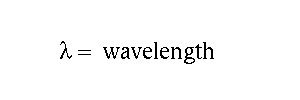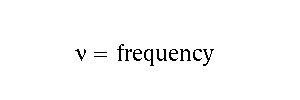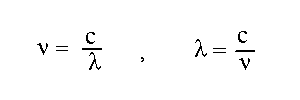# The relation between wavelength and frequency

Light moves with a speed

c = 3 x 108 m/s.

We denote wavelength byFrequency is how many complete waves go by per second. We denote frequency byFrequency is measured in Hertz = Hz = 1/seconds.

If a wave with a wavelength of 2 meters is going by at a speed of 6 meters/second, then 3 complete waves go by in 1 second. That is, the frequency is 6/2 = 3 waves/second, or 3 Hz. (See units ).ThusFor example, radio station KUGN broadcasts at a frequency of 590 KHz. What is the wavelength of the radio waves?

wavelength
= c/frequency
= (3 x 108 m/s)/(590 x 103 Hz)
= (3 x 108)/(0.590 x 106) m
~ 5 x 102 m
= 500 m.

Updated 1 October 2007

Davison E. Soper, Institute of Theoretical Science, University of Oregon, Eugene OR 97403 USA